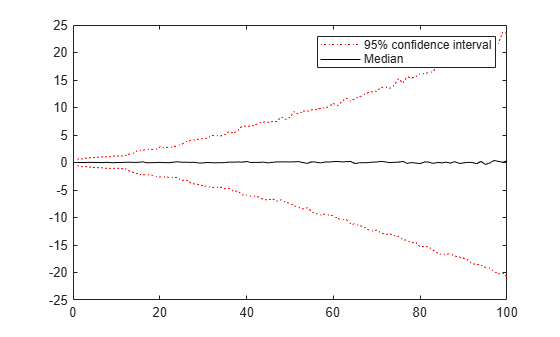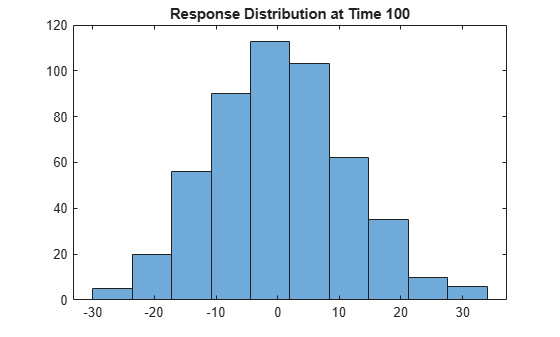simulate

Monte Carlo simulation of ARIMA or ARIMAX models

Syntax

[Y,E] = simulate(Mdl,numObs)
[Y,E,V] = simulate(Mdl,numObs)
[Y,E,V] = simulate(Mdl,numObs,Name,Value)

Description

[Y,E] = simulate(Mdl,numObs) simulates sample paths and innovations from the ARIMA model, Mdl. The responses can include the effects of seasonality.

[Y,E,V] = simulate(Mdl,numObs) additionally simulates conditional variances, V.

[Y,E,V] = simulate(Mdl,numObs,Name,Value) simulates sample paths with additional options specified by one or more Name,Value pair arguments.

Input Arguments

 Mdl ARIMA or ARIMAX model, specified as an arima model returned by arima or estimate. The properties of Mdl cannot contain NaNs. numObs Positive integer that indicates the number of observations (rows) to generate for each path of the outputs Y, E, and V.

Name-Value Arguments

Specify optional comma-separated pairs of Name,Value arguments. Name is the argument name and Value is the corresponding value. Name must appear inside quotes. You can specify several name and value pair arguments in any order as Name1,Value1,...,NameN,ValueN.

 E0 Mean zero presample innovations that provide initial values for the model. E0 is a column vector or a matrix with at least NumPaths columns and enough rows to initialize the model and any conditional variance model. The number of observations required is at least Mdl.Q, but can be more if you specify a conditional variance model. If the number of rows exceeds the number necessary, then simulate only uses the most recent observations. If the number of columns exceeds NumPaths, then simulate only uses the first NumPaths columns. If E0 is a column vector, then it is applied to each simulated path. The last row contains the most recent presample observation. Default: simulate sets the necessary presample observations to 0. NumPaths Positive integer that indicates the number of sample paths (columns) to generate. Default: 1 V0 Positive presample conditional variances which provide initial values for any conditional variance model. If the variance of the model is constant, then V0 is unnecessary. V0 is a column vector or a matrix with at least NumPaths columns and enough rows to initialize the variance model. If the number of rows exceeds the number necessary, then simulate only uses the most recent observations. If the number of columns exceeds NumPaths, then simulate only uses the first NumPaths columns. If V0 is a column vector, then simulate applies it to each simulated path. The last row contains the most recent observation. Default: simulate sets the necessary presample observations to the unconditional variance of the conditional variance process. X Matrix of predictor data with length Mdl.Beta columns of separate series. The number of observations (rows) of X must equal or exceed numObs. If the number of observations of X exceeds numObs, then simulate only uses the most recent observations. simulate applies the entire matrix X to each simulated response series. The last row contains the most recent observation. Default: simulate does not use a regression component regardless of the value of Mdl.Beta. Y0 Presample response data that provides initial values for the model. Y0 is a column vector or a matrix with at least Mdl.P rows and NumPaths columns. If the number of rows exceeds Mdl.P, then simulate only uses the most recent Mdl.P observations. If the number of columns exceeds NumPaths, then simulate only uses the first NumPaths columns. If Y0 is a column vector, then it is applied to each simulated path. The last row contains the most recent presample observation. Default: simulate sets the necessary presample observations to the unconditional mean if the AR process is stable, or to 0 for unstable processes or when you specify X.

Notes

• NaNs indicate missing values, and simulate removes them. The software merges the presample data, then uses list-wise deletion to remove any NaNs in the presample data matrix or X. That is, simulate sets PreSample = [Y0 E0 V0], then it removes any row in PreSample or X that contains at least one NaN.

• The removal of NaNs in the main data reduces the effective sample size. Such removal can also create irregular time series.

• simulate assumes that you synchronize the predictor series such that the most recent observations occur simultaneously. The software also assumes that you synchronize the presample series similarly.

Output Arguments

 Y numObs-by-NumPaths matrix of simulated response data. E numObs-by-NumPaths matrix of simulated mean zero innovations. V numObs-by-NumPaths matrix of simulated conditional variances of the innovations in E.

Examples

expand all

Simulate response and innovation paths from a multiplicative seasonal model.

Specify the model

$\left(1-L\right)\left(1-{L}^{12}\right){y}_{t}=\left(1-0.5L\right)\left(1+0.3{L}^{12}\right){\epsilon }_{t},$

where ${\epsilon }_{t}$ follows a Gaussian distribution with mean 0 and variance 0.1.

Mdl = arima('MA',-0.5,'SMA',0.3,...
'SMALags',12,'D',1,'Seasonality',12,...
'Variance',0.1,'Constant',0);

Simulate 500 paths with 100 observations each.

rng default % For reproducibility
[Y,E] = simulate(Mdl,100,'NumPaths',500);

figure
subplot(2,1,1);
plot(Y)
title('Simulated Response')

subplot(2,1,2);
plot(E)
title('Simulated Innovations')Plot the 2.5th, 50th (median), and 97.5th percentiles of the simulated response paths.

lower = prctile(Y,2.5,2);
middle = median(Y,2);
upper = prctile(Y,97.5,2);

figure
plot(1:100,lower,'r:',1:100,middle,'k',...
1:100,upper,'r:')
legend('95% Interval','Median')Compute statistics across the second dimension (across paths) to summarize the sample paths.

Plot a histogram of the simulated paths at time 100.

figure
histogram(Y(100,:),10)
title('Response Distribution at Time 100')Simulate three predictor series and a response series.

Specify and simulate a path of length 20 for each of the three predictor series modeled by

$\left(1-0.2L\right){x}_{it}=2+\left(1+0.5L-0.3{L}^{2}\right){\eta }_{it},$

where ${\eta }_{it}$ follows a Gaussian distribution with mean 0 and variance 0.01, and $i$ = {1,2,3}.

[MdlX1,MdlX2,MdlX3] = deal(arima('AR',0.2,'MA',...
{0.5,-0.3},'Constant',2,'Variance',0.01));

rng(4); % For reproducibility
simX1 = simulate(MdlX1,20);
simX2 = simulate(MdlX2,20);
simX3 = simulate(MdlX3,20);
SimX = [simX1 simX2 simX3];

Specify and simulate a path of length 20 for the response series modeled by

$\left(1-0.05L+0.02{L}^{2}-0.01{L}^{3}\right)\left(1-L{\right)}^{1}{y}_{t}=0.05+{x}_{t}^{\prime }\left[\begin{array}{c}0.5\\ -0.03\\ -0.7\end{array}\right]+\left(1+0.04L+0.01{L}^{2}\right){\epsilon }_{t},$

where ${\epsilon }_{t}$ follows a Gaussian distribution with mean 0 and variance 1.

MdlY = arima('AR',{0.05 -0.02 0.01},'MA',...
{0.04,0.01},'D',1,'Constant',0.5,'Variance',1,...
'Beta',[0.5 -0.03 -0.7]);
simY = simulate(MdlY,20,'X',SimX);

Plot the series together.

figure
plot([SimX simY])
title('Simulated Series')
legend('{X_1}','{X_2}','{X_3}','Y')Forecast the daily NASDAQ Composite Index using Monte Carlo simulations.

Load the NASDAQ data included with the toolbox. Extract the first 1500 observations for fitting.

nasdaq = DataTable.NASDAQ(1:1500);
n = length(nasdaq);

Specify, and then fit an ARIMA(1,1,1) model.

NasdaqModel = arima(1,1,1);
NasdaqFit = estimate(NasdaqModel,nasdaq);

ARIMA(1,1,1) Model (Gaussian Distribution):

Value      StandardError    TStatistic      PValue
_________    _____________    __________    __________

Constant      0.43031       0.18555           2.319       0.020393
AR{1}       -0.074392      0.081985        -0.90739         0.3642
MA{1}         0.31126      0.077266          4.0284     5.6154e-05
Variance       27.826       0.63625          43.735              0

Simulate 1000 paths with 500 observations each. Use the observed data as presample data.

rng default;
Y = simulate(NasdaqFit,500,'NumPaths',1000,'Y0',nasdaq);

Plot the simulation mean forecast and approximate 95% forecast intervals.

lower = prctile(Y,2.5,2);
upper = prctile(Y,97.5,2);
mn = mean(Y,2);

figure
plot(nasdaq,'Color',[.7,.7,.7])
hold on
h1 = plot(n+1:n+500,lower,'r:','LineWidth',2);
plot(n+1:n+500,upper,'r:','LineWidth',2)
h2 = plot(n+1:n+500,mn,'k','LineWidth',2);

legend([h1 h2],'95% Interval','Simulation Mean',...
'Location','NorthWest')
title('NASDAQ Composite Index Forecast')
hold offReferences

 Box, G. E. P., G. M. Jenkins, and G. C. Reinsel. Time Series Analysis: Forecasting and Control 3rd ed. Englewood Cliffs, NJ: Prentice Hall, 1994.

 Enders, W. Applied Econometric Time Series. Hoboken, NJ: John Wiley & Sons, 1995.

 Hamilton, J. D. Time Series Analysis. Princeton, NJ: Princeton University Press, 1994.